# KS1 Maths Quiz - Year 2 Patterns and Sequences (Questions)

This quiz addresses the requirements of the National Curriculum KS1 Maths and Numeracy for children aged 6 and 7 in year 2. Specifically this quiz is aimed at the section dealing with recognising and extending patterns and sequences.

When talking about patterns and sequences, children in Year 2 should be familiar with sequences which increase or decrease in 2s, 5s or 10s and be able to extend them. They can then use this knowledge in order to work out the rule for more complex sequences and extend them also, or perhaps even begin to make their own patterns and sequences which follow a rule.

1.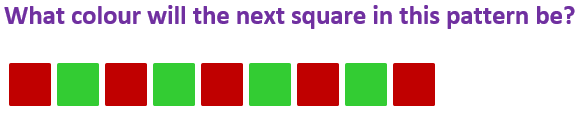[ ] Red [ ] Green [ ] Blue [ ] Orange
2.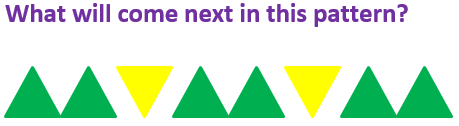[ ] Two yellow triangles [ ] A green triangle, upside down [ ] A green triangle [ ] A yellow triangle, upside down
3.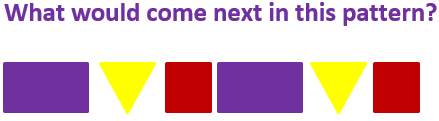[ ] A purple rectangle [ ] A yellow triangle [ ] A green triangle [ ] A red square
4.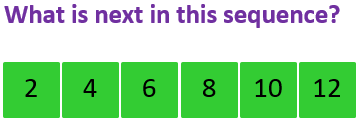[ ] 20 [ ] 14 [ ] 24 [ ] 15
5.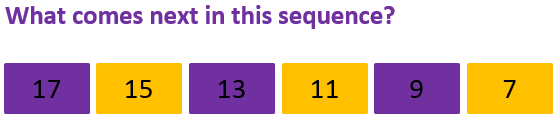[ ] A yellow rectangle with the number 8 [ ] A purple rectangle with the number 3 [ ] A yellow rectangle with the number 6 [ ] A purple rectangle with the number 5
6.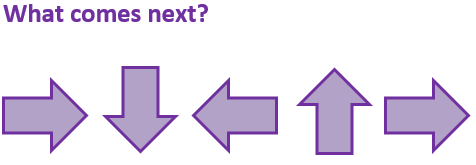[ ] An arrow pointing down [ ] An arrow pointing left [ ] An arrow pointing right [ ] An arrow pointing up
7.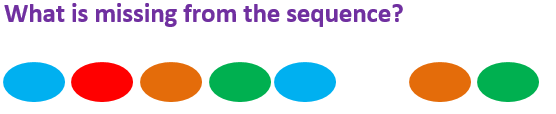[ ] A purple oval [ ] A green oval [ ] An orange oval [ ] A red oval
8.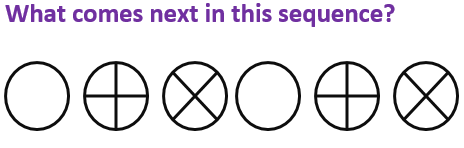[ ] A circle containing + [ ] A circle containing x [ ] A blank circle [ ] A triangle
9.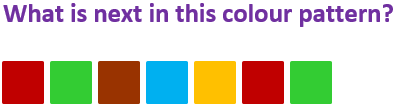[ ] A red square [ ] A green square [ ] A blue square [ ] A brown square
10.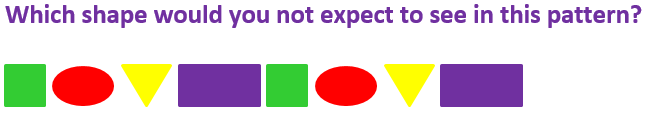[ ] A triangle [ ] A square [ ] An oval [ ] A starKS1 Maths Quiz - Year 2 Patterns and Sequences (Answers)
1.[ ] Red [x] Green [ ] Blue [ ] Orange
This is a simple repeating pattern of red and green
2.[ ] Two yellow triangles [ ] A green triangle, upside down [ ] A green triangle [x] A yellow triangle, upside down
Sometimes the same shape is used, but in different positions
3.[x] A purple rectangle [ ] A yellow triangle [ ] A green triangle [ ] A red square
Some patterns are more complex and use more shapes and colours
4.[ ] 20 [x] 14 [ ] 24 [ ] 15
The numbers are all even and are going up in twos
5.[ ] A yellow rectangle with the number 8 [ ] A purple rectangle with the number 3 [ ] A yellow rectangle with the number 6 [x] A purple rectangle with the number 5
This pattern has two different parts: the changing colour and the numbers
6.[x] An arrow pointing down [ ] An arrow pointing left [ ] An arrow pointing right [ ] An arrow pointing up
The arrows are turning round in a clockwise movement
7.[ ] A purple oval [ ] A green oval [ ] An orange oval [x] A red oval
Sometimes it’s useful to say the colours aloud to hear the missing one
8.[ ] A circle containing + [ ] A circle containing x [x] A blank circle [ ] A triangle
This repeating pattern uses three different versions of a circle
9.[ ] A red square [ ] A green square [ ] A blue square [x] A brown square
This pattern uses five different colours!
10.[ ] A triangle [ ] A square [ ] An oval [x] A star
A star does not feature in this pattern